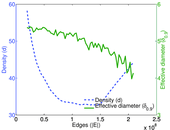# Wikipedia edits (en)

This is the bipartite edit network of the English Wikipedia. It contains users and pages from the English Wikipedia, connected by edit events. Each edge represents an edit. The dataset includes the timestamp of each edit.

 Code `en` Internal name `edit-enwiki` Name Wikipedia edits (en) Data source http://dumps.wikimedia.org/ AvailabilityDataset is available for download Consistency check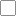Check was not executed Category Authorship network Dataset timestamp 2017-10-20 Node meaning User, article Edge meaning Edit Network formatBipartite, undirected Edge typeUnweighted, multiple edges Temporal dataEdges are annotated with timestamps

## Statistics

 Size n = 50,757,442 Left size n1 = 8,116,897 Right size n2 = 42,640,545 Volume m = 572,591,272 Unique edge count m̿ = 255,709,661 Wedge count s = 6,299,863,981,390 Maximum degree dmax = 5,576,228 Maximum left degree d1max = 5,576,228 Maximum right degree d2max = 1,275,383 Average degree d = 22.561 9 Average left degree d1 = 70.543 1 Average right degree d2 = 13.428 3 Fill p = 1.486 20 × 10−6 Average edge multiplicity m̃ = 2.239 22 Size of LCC N = 49,109,194 Diameter δ = 16 50-Percentile effective diameter δ0.5 = 3.718 73 90-Percentile effective diameter δ0.9 = 4.833 83 Mean distance δm = 4.293 10 Gini coefficient G = 0.890 462 Balanced inequality ratio P = 0.114 005 Left balanced inequality ratio P1 = 0.059 418 7 Right balanced inequality ratio P2 = 0.154 300 Relative edge distribution entropy Her = 0.790 635 Power law exponent γ = 2.213 56 Degree assortativity ρ = −0.027 743 0 Degree assortativity p-value pρ = 0.000 00 Spectral norm α = 308,364

## Plots

### Degree distribution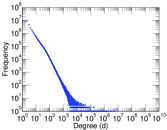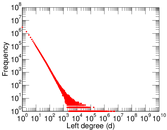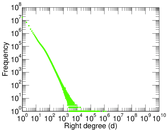### Cumulative degree distribution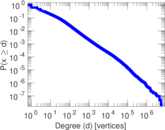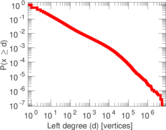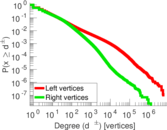### Lorenz curve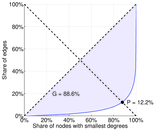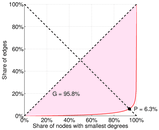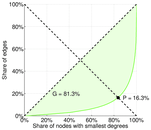### Spectral distribution of the adjacency matrix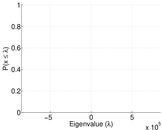### Spectral distribution of the normalized adjacency matrix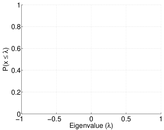### Spectral distribution of the Laplacian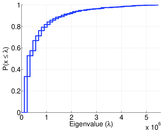### Spectral graph drawing based on the adjacency matrix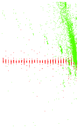### Spectral graph drawing based on the normalized adjacency matrix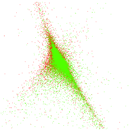### Degree assortativity### Hop distribution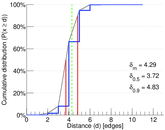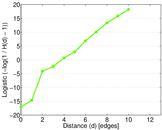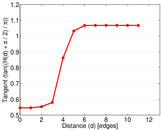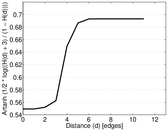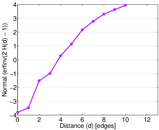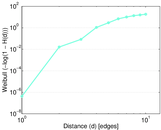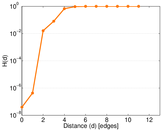### Edge weight/multiplicity distribution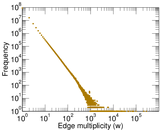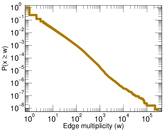### Temporal distribution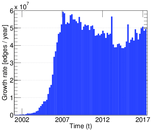### Diameter/density evolution